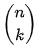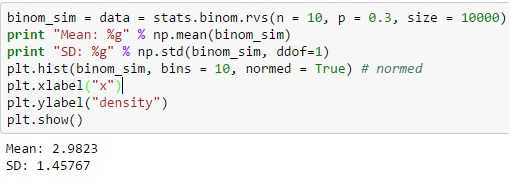HomeBlogData ScienceBinomial Distribution in Python with Real World Examples 

# Binomial Distribution in Python with Real World Examples 

Last updated:
8th Jan, 2021
Views
6 MinsView AllThe value of probability and statistics in the field of data science has been immense, with artificial intelligence and machine learning relying heavily on them. We are using process models of normal distribution every time we conduct A/B testing and investment modeling.

However, the binomial distribution in Python gets applied in multiple ways to carry out several processes. But, before getting started with binomial distribution in Python, you need to know about binomial distribution in general and its use in everyday life. If you are a beginner and interested to learn more about data science, check out our data science training from top universities.

## What is the Binomial Distribution?

Have you ever flipped a coin? If you have, then you must know about the probability of getting heads or tails is equal. But, how about the likelihood of getting seven tails in total ten flips of a coin? This is where binomial distribution can help in calculating each flip’s results, and thus finding out the probability of getting seven tails for ten flips of a coin.

The crux of probability distribution comes from the variance of any event. For each ten coin tosses set, the probability of getting heads and tails can be anywhere between one to ten times, equally and likely. The uncertainty in the result (also known as variance) helps in generating the distribution of the outcomes produced.

In other words, the binomial distribution is a process where there are only two possible outcomes: true or false. Therefore, it has an equal probability of both the results across all events, as the same actions are performed each time. There is only one condition… The steps need to be completely unaffected of each other, and the results may or may not be equally likely.

Therefore, the probability function of a binomial distribution is:

ff(kk,nn,pp) =P rPr(kk;nn,pp) = P rPr (XX=kk)  =Source

Where,=nn!kk!(nn!-kk!)

Here, n = total number of trials

p = success probability

k = target number of successes

## Binomial Distribution in Python

For binomial distribution via Python, you can produce the distinct random variable from the binom.rvs () function, where ‘n’ is defined as the total frequency of trials, and ‘p’ is equal to success probability.

You can also move the distribution using the loc function, and the size defines the frequency of an action that gets repeated in the series. Adding a random_state can help in maintaining reproducibility.Source

## Real-world Examples of Binomial Distribution in Python

upGrad’s Exclusive Data Science Webinar for you –

There are many more events (bigger than coin tosses) that can get addressed by binomial distribution in Python. Some of the use cases can help track and improve ROI (return on investments) for big and small companies. Here’s how:

• Think about a call center where each employee gets assigned with 50 calls each day on an average.
• The probability of conversion over each call is equal to 4%.
• The average revenue generation for the company based on each such conversion is that of USD 20.
• If you analyze 100 such employees, who get paid USD 200 each day, then

n = 50

p = 4%

## Explore our Popular Data Science Courses

 Executive Post Graduate Programme in Data Science from IIITB Professional Certificate Program in Data Science for Business Decision Making Master of Science in Data Science from University of Arizona Advanced Certificate Programme in Data Science from IIITB Professional Certificate Program in Data Science and Business Analytics from University of Maryland Data Science Courses

The code can generate output as following:

• Average conversion rate for each employee = 2.13
• The standard deviation of conversions for each call center personnel = 1.48
• Gross conversion = 213
• Gross revenue generation = USD 21,300
• Gross expense = USD 20,000
• Gross profits = USD 1,300

Binomial distribution models and other probability distributions can only predict an approximation that can get close to the real-world in terms of the action parameters, ‘n’ and ‘p’. It helps us to understand and identify our focus areas and improve the overall chances of better performance and effectiveness.

## Read our popular Data Science Articles

 Data Science Career Path: A Comprehensive Career Guide Data Science Career Growth: The Future of Work is here Why is Data Science Important? 8 Ways Data Science Brings Value to the Business Relevance of Data Science for Managers The Ultimate Data Science Cheat Sheet Every Data Scientists Should Have Top 6 Reasons Why You Should Become a Data Scientist A Day in the Life of Data Scientist: What do they do? Myth Busted: Data Science doesn’t need Coding Business Intelligence vs Data Science: What are the differences?

## Top Data Science Skills to Learn

 Top Data Science Skills to Learn 1 Data Analysis Course Inferential Statistics Courses 2 Hypothesis Testing Programs Logistic Regression Courses 3 Linear Regression Courses Linear Algebra for Analysis

If you are curious to learn about data science, check out IIIT-B & upGrad’s Executive PG Programme in Data Science which is created for working professionals and offers 10+ case studies & projects, practical hands-on workshops, mentorship with industry experts, 1-on-1 with industry mentors, 400+ hours of learning and job assistance with top firms.#### Rohit Sharma

Blog Author
Rohit Sharma is the Program Director for the UpGrad-IIIT Bangalore, PG Diploma Data Analytics Program.
Get Free Consultation

Select CourseSelectBy clicking 'Submit' you Agree to

#### Data Science Skills to Master

1What is the difference between discrete probability distribution and continuous probability distribution?

The discrete probability distribution or simply discrete distribution calculates the probabilities of a random variable that can be discrete. For example, if we toss a coin twice, the probable values of a random variable X that denotes the total number of heads will be {0, 1, 2} and not any random value. Bernoulli, Binomial, Hypergeometric are some examples of the discrete probability distribution. On the other hand, the continuous probability distribution provides the probabilities of a random value that can be any random number. For example, the value of a random variable X that denotes the height of citizens of a city could be any number like 161.2, 150.9, etc. Normal, Student’s T, Chi-square are some of the examples of continuous distribution.

2What is the significance of probability in data science?

As data science is all about studying data, probability plays a key role here. The following reasons describe how probability is an indispensable part of data science: It helps analysts and researchers make predictions out of data sets. These kinds of estimated results are the foundation for further analysis of the data. Probability is also used while developing algorithms used in machine learning models. It helps in analyzing the data sets used for training the models. It allows you to quantify data and derive results such as derivatives, mean, and distribution. All the results achieved using probability eventually summarizes the data. This summary also helps in the identification of existing outliers in the data sets.

3Explain hypergeometric distribution. In what case it tends to be binomial distribution?

successes over the number of trials without any replacement. Let us say we have a bag full of red and green balls and we have to find the probability of picking a green ball in 5 attempts but each time we pick a ball, we do not return it back to the bag. This is an apt example of the hypergeometric distribution.
For larger N, it is very difficult to calculate the hypergeometric distribution but when N is small, it tends to the binomial distribution in this case.

## Suggested Blogs

46813
As a working professional, you are familiar with terms like data, database, information, processing, etc. You must have also come across terms like da